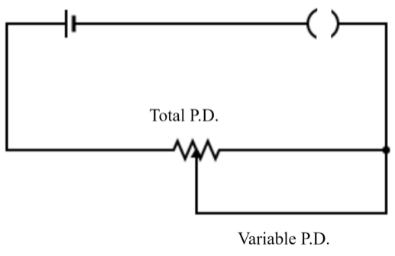Courses
Courses for Kids
Free study material
Offline Centres
MoreLast updated date: 30th Nov 2023
Total views: 277.5k
Views today: 7.77k

# The arrangement as shown in the figure is called as ?Verified
277.5k+ views
Hint: In the given diagram there is a voltage source, a key to close or open the circuit and a resistor. Also, there is one more wire which is connecting the circuit to a point that is somewhere in the middle of the resistor, which is labeled as ‘variable P.D’.

Suppose many resistors are connected in series with each other and then to a voltage source, then the voltage across all of them gets divided in such a way that the voltage across any resistor ${{R}_{x}}$ is given by: ${{V}_{x}}(t)=V(t)\dfrac{{{R}_{x}}}{{{R}_{T}}}$, where ${{R}_{T}}$ is the total resistance of the series combination. Voltage dividers are used for making potentiometers; in fact, they are also used in a wheat-stone bridge circuit and multimeter circuit.